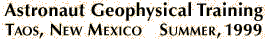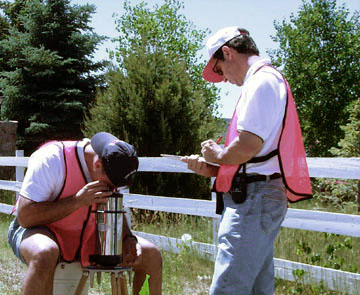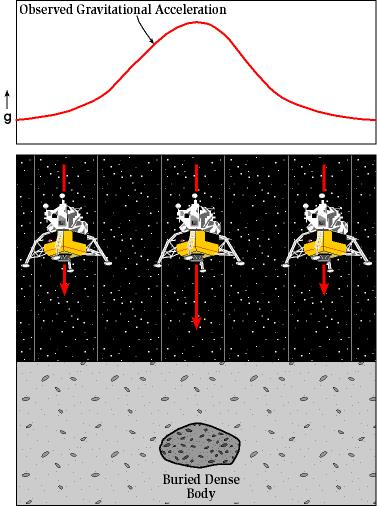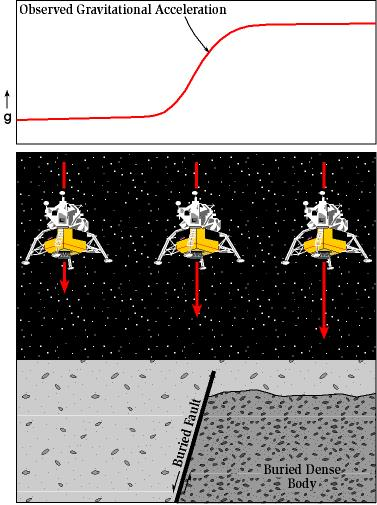#####Penguin PoweredA joint project between NASA/JSC & the NM Bureau of Geology

## The Gravity Geophysical MethodThe Worden gravimeter is entirely mechanical and optical relying only on an AA battery for illuminating the crosshairs. It uses a fixed length spring and mass attached to a calibration spring and vernier scale to measure gravitational acceleration.

For human exploration of the solar system, instruments must meet criteria of low mass, low volume, low power demand, safe operation, and ruggedness and reliability (Meyer et al., 1995; Hoffman, 1997; Budden, 1999). Tools used for planetary exploration will need to address fundamental scientific questions and identify precious resources, such as water.

The primary goal of studying detailed gravity data is to provide a better understanding of the subsurface geology. The gravity method is a relatively cheap, non-invasive, non-destructive remote sensing method that has already been tested on the lunar surface. It is also passive – that is, no energy need be put into the ground in order to acquire data; thus, the method is well suited to a populated setting such as Taos, and a remote setting such as Mars. The small portable instrument also permits walking traverses – ideal, in view of the congested tourist traffic in Taos.

Measurements of gravity provide information about densities of rocks underground. There is a wide range in density among rock types, and therefore geologists can make inferences about the distribution of strata. In the Taos Valley, we are attempting to map subsurface faults. Because faults commonly juxtapose rocks of differing densities, the gravity method is an excellent exploration choice.

Gravity Survey - Measurements of the gravitational field at a series of different locations over an area of interest. The objective in exploration work is to associate variations with differences in the distribution of densities and hence rock types.

(Definition from the Encyclopedic Dictionary of Exploration Geophysics by R. E. Sheriff, published by the Society of Exploration Geophysics).Cartoon illustrations showing the relative surface variation of gravitational acceleration over geologic structures.

### This is a generalized summary of the types of corrections that we have applied to the Taos gravity data

The Gal (for Galileo) is the cgs unit for acceleration where one Gal equals 1 centimenter per second squared. Because variations in gravity are very small, units for gravity surveys are generally in milligals (mGal) where 1 mGal is one thousandth of 1cm/s2. Standard gravity ( gnor g0 ) is taken as the freefall accelleration of an object at sea level at a latitude of 45.5° and is 980.665 cm/s2 (or equivalently 9.80665 m/s2). Standard gravity is therefore 980.665 Gal or 980665 mGal. It is useful to remember that 1 mGal is just a bit more than 1 millionth of gn (1.01972 x 10-6 gn).

Observed Gravity (gobs ) - Gravity readings observed at each gravity station after corrections have been applied for instrument drift and earth tides.

Latitude Correction (gn ) - Correction subtracted from gobs that accounts for Earth's elliptical shape and rotation. The gravity value that would be observed if Earth were a perfect (no geologic or topographic complexities), rotating ellipsoid is referred to as the normal gravity.

gn = 978031.85 (1.0 + 0.005278895 sin2(lat) + 0.000023462 sin4(lat)) (mGal)

where lat is latitude

Free Air Corrected Gravity (gfa ) - The free-air correction accounts for gravity variations caused by elevation differences in the observation locations. The form of the Free-Air gravity anomaly, gfa , is given by:

gfa = gobs - gn+ 0.3086h (mGal)

where h is the elevation (in meters) at which the gravity station is above the datum (typically sea level).

Bouguer Slab Corrected Gravity (gb ) - The Bouguer correction is a first-order correction to account for the excess mass underlying observation points located at elevations higher than the elevation datum (sea level or the geoid). Conversely, it accounts for a mass deficiency at observation points located below the elevation datum. The form of the Bouguer gravity anomaly, gb, is given by:

gb = gobs - gn + 0.3086h - 0.04193r h (mGal)

where r is the average density of the rocks underlying the survey area.

Terrain Corrected Bouguer Gravity (gt ) - The Terrain correction accounts for variations in the observed gravitational acceleration caused by variations in topography near each observation point. Because of the assumptions made during the Bouguer Slab correction, the terrain correction is positive regardless of whether the local topography consists of a mountain or a valley. The form of the Terrain corrected, Bouguer gravity anomaly, gt , is given by:

gt = gobs - gn + 0.3086h - 0.04193r h + TC (mGal)

where TC is the value of the computed Terrain correction.

Assuming these corrections have accurately accounted for the variations in gravitational acceleration they were intended to account for, any remaining variations in the gravitational acceleration associated with the Terrain Corrected Bouguer Gravity can be assumed to be caused by geologic structure.

Follow the links to see how these corrections affect the gravity profiles for each of the survey lines.
G1 | G2 | G3 | G4 | G5 | G6 | G7

 Main | Previous | Next comments/questions to Paul Bauer